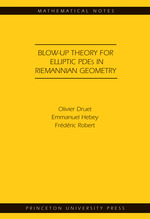# Blow-up Theory for Elliptic PDEs in Riemannian Geometry (MN-45):

Olivier Druet
Emmanuel Hebey
Frédéric Robert
Pages: 224
https://www.jstor.org/stable/j.ctt7s38w

1. Front Matter
(pp. i-iv)
(pp. v-vi)
3. Preface
(pp. vii-viii)
The Authors
4. Chapter One Background Material
(pp. 1-12)

We recall in this chapter basic facts concerning Riemannian geometry and nonlinear analysis on manifolds. For reasons of length, we are obliged to be succinct and partial. Possible references are Chavel , do Carmo , Gallot-Hulin-Lafontaine , Hebey , Jost , Kobayashi-Nomizu , Sakai , and Spivak . As a general remark, we mention that Einstein’s summation convention is adopted: an index occurring twice in a product is to be summed. This also holds for the rest of this book.

We start with a few notions in differential geometry. LetMbe a Hausdorff topological space. We say thatM...

5. Chapter Two The Model Equations
(pp. 13-24)

Let$\left( {M,g} \right)$be a smooth compact Riemannian manifold of dimensionn ≥ 3. We let$\left( {{h_\alpha }} \right)$be a sequence of smooth functions onM, and consider equations like.

${\Delta _g}u + {h_\alpha }u = {u^{2* - 1}}$$\left( {E{}_\alpha } \right)$

where${\Delta _g} = - di{v_g}\nabla$is the Laplace-Beltrami operator,$2{\kern 1pt} * = 2n/(n\; - \;2)$is the critical Sobolev exponent for the embedding of the Sobolev space$H_1^2\left( M \right)$into Lebesgue’s spaces${L^p}(M)$, anduis required to be positive. By standard regularity theory, as developed by Gilbarg-Trudinger , and thanks to the maximum principle, if$u \in H_1^2\left( M \right)$is a nonnegative solution of$\left( {{E_\alpha }} \right)$, thenuis smooth and either$u > 0$everywhere or$u \equiv 0$. As already mentioned, thanks to the...

6. Chapter Three Blow-up Theory in Sobolev Spaces
(pp. 25-50)

An important result of the 1980s describes the asymptotic behavior of Palais-Smale sequences associated with equations like

$\Delta u = {u^{2*\, - \,1}}$, (3.0.1)

where$\Delta$is the Euclidean Laplacian, anduis required to vanish on the boundary of a smooth bounded open subset$\Omega$of the Euclidean space${\mathbb{R}^n}$. This result was proved by Struwe . Related references are Brézis , Brézis-Coron [12, 13], Lions , Sacks-Uhlenbeck , Schoen , and Wente . Let$D_1^2\left( {{\mathbb{R}^n}} \right)$be the homogeneous Sobolev space de ned as the completion of$C_0^\infty \left( {{\mathbb{R}^n}} \right)$, the space of smooth functions with compact support in${\mathbb{R}^n}$, with respect to the norm a...

7. Chapter Four Exhaustion and Weak Pointwise Estimates
(pp. 51-66)

Let$\left({M,g}\right)$be a smooth compact Riemannian manifold of dimension$n\ge3$. We let$({h_\alpha})$be a sequence of smooth functions onM, and consider equations like

${\Delta_g}u\;+\;{h_\alpha}u={u^{2*\,-\;1}}\quad\quad\quad\quad\quad({E_\alpha})$

whereuis required to be positive. We let$({u_\alpha})$be a sequence of solutions to$({E_\alpha})$, so that${u_\alpha}>0$and

${\Delta_g}{u_\alpha}+\;{h_\alpha}{u_\alpha}=u_\alpha^{2*\,-\;1}$

for all$\alpha$. We describe in this chapter an exhaustion method for the blow-up behavior of the${u_\alpha}$’s. This method provides a constructive approach to Theorem 3.1, and weak (with respect to the material in Chapter 5 and 6) pointwise estimates on the${u_\alpha}$’s.

We assume in what follows that there...

8. Chapter Five Asymptotics When the Energy Is of Minimal Type
(pp. 67-82)

Let$\left({M,g}\right)$be a smooth compact Riemannian manifold of dimension$n\ge\;3$. We let$({h_\alpha})$be a sequence of smooth functions onM, and consider equations like

${\Delta_g}u\;+\;{h_\alpha}u\;=\;{u^{2*\,-\,1}}\quad\quad\quad\quad\quad\quad({E_\alpha})$

whereuis required to be positive. We let$({u_\alpha})$be a sequence of solutions to$({E_\alpha})$, so that${u_\alpha}>\;0$and

${\Delta_g}{u_\alpha}+\;{h_\alpha}{u_\alpha}=u_\alpha^{2*\,-1}$

for all$\alpha$. For the reader’s convenience, we describe in this chapter the${C^0}$-theory for the blow-up behavior of the${u_\alpha}$’s when the energy of the${u_\alpha}$’s is of minimal type. The general situation of arbitrary energies is treated in the next chapter.

As in Chapter 4, we assume in what follows...

9. Chapter Six Asymptotics When the Energy Is Arbitrary
(pp. 83-200)

Let$\left({M,g}\right)$be a smooth compact Riemannian manifold of dimension$n\ge\;3$. We let$({h_\alpha})$be a sequence of smooth functions onMand consider equations like

${\Delta_g}u\;+\;{h_\alpha}u\;=\;{u^{2*\,-\,1}}\quad\quad\quad\quad\quad\quad({E_\alpha})$

whereuis required to be positive. We let$({u_\alpha})$be a sequence of solutions to$({E_\alpha})$, so that${u_\alpha}>0$and

${\Delta_g}{u_\alpha}+\;{h_\alpha}{u_\alpha}=u_\alpha^{2*\,-1}$

for all$\alpha$. We describe in this chapter the${C^0}$-theory for the blow-up behavior of the${u_\alpha}$’s when the energy of the${u_\alpha}$’s is arbitrary.

As in Chapters 4 and 5, we assume in what follows that there exist$0<\theta<1$and a smooth (or only${C^{0,\theta}}$) function${h_\infty}$onMsuch that

the...

10. Appendix A. The Green’s Function on Compact Manifolds
(pp. 201-208)
11. Appendix B. Coercivity Is a Necessary Condition
(pp. 209-212)
12. Bibliography
(pp. 213-218)